# 手把手：基于概率编程Pyro的金融预测，让正则化结果更有趣！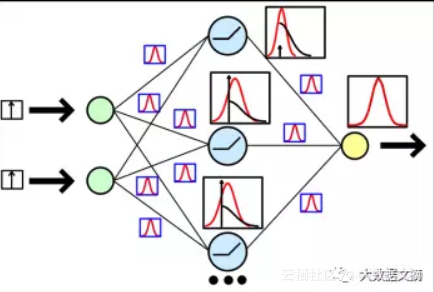Hi！又见面啦。去年我推出了几篇基于神经网络的金融预测教程，我认为有些结果还是蛮有趣的，值得应用在实际交易中。

• 模式识别和机器学习：

http://www.springer.com/us/book/9780387310732

• 为黑客设计的贝叶斯方法：

• PyMC3：

https://github.com/pymc-devs/pymc3

• Edward：

http://edwardlib.org/

• Pyro：

http://pyro.ai/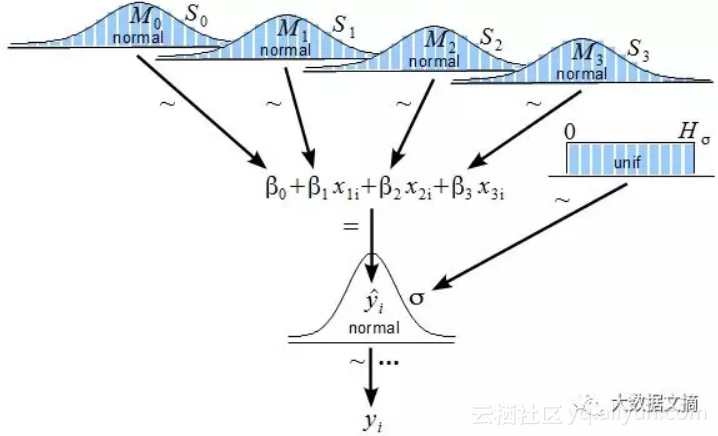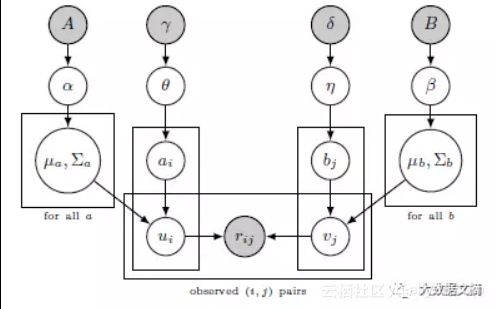https://bitinfocharts.com/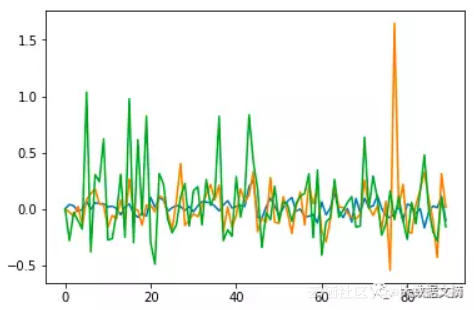Pyro官方教程：

http://pyro.ai/examples/bayesian_regression.html

class RegressionModel(nn.Module):

def __init__(self, p):
super(RegressionModel, self).__init__()

self.linear = nn.Linear(p, 1)

def forward(self, x):
# x * w + b
return self.linear(x)

def model(data):

# Create unit normal priors over the parameters

mu = Variable(torch.zeros(1, p)).type_as(data)

sigma = Variable(torch.ones(1, p)).type_as(data)

bias_mu = Variable(torch.zeros(1)).type_as(data)

bias_sigma = Variable(torch.ones(1)).type_as(data)

w_prior, b_prior = Normal(mu, sigma), Normal(bias_mu, bias_sigma)

priors = {'linear.weight': w_prior, 'linear.bias': b_prior}

lifted_module = pyro.random_module("module", regression_model, priors)

lifted_reg_model = lifted_module()

with pyro.iarange("map", N, subsample=data):

x_data = data[:, :-1]

y_data = data[:, -1]

# run the regressor forward conditioned on inputs

prediction_mean = lifted_reg_model(x_data).squeeze()

pyro.sample("obs",

Normal(prediction_mean, Variable(torch.ones(data.size(0))).type_as(data)),

obs=y_data.squeeze())

def guide(data):

w_log_sig = Variable(0.1 * torch.ones(1, p).type_as(data.data), requires_grad=True)

b_log_sig = Variable(0.1 * torch.ones(1).type_as(data.data), requires_grad=True)

mw_param = pyro.param("guide_mean_weight", w_mu)

sw_param = softplus(pyro.param("guide_log_sigma_weight", w_log_sig))

mb_param = pyro.param("guide_mean_bias", b_mu)

sb_param = softplus(pyro.param("guide_log_sigma_bias", b_log_sig))

w_dist = Normal(mw_param, sw_param)

b_dist = Normal(mb_param, sb_param)

dists = {'linear.weight': w_dist, 'linear.bias': b_dist}

lifted_module = pyro.random_module("module", regression_model, dists)
return lifted_module()

（~Normal(0, 0.1)）

for j in range(3000):

epoch_loss = 0.0

perm = torch.randperm(N)

# shuffle data

data = data[perm]

# get indices of each batch

all_batches = get_batch_indices(N, 64)
for ix, batch_start in enumerate(all_batches[:-1]):

batch_end = all_batches[ix + 1]

batch_data = data[batch_start: batch_end]

epoch_loss += svi.step(batch_data)

preds = []
for i in range(100):

sampled_reg_model = guide(X_test)

pred = sampled_reg_model(X_test).data.numpy().flatten()

preds.append(pred)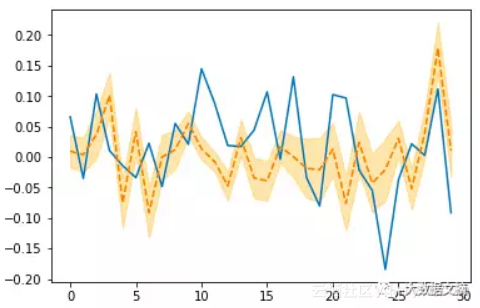30天的贝叶斯模型预测

def get_model(input_size):

main_input = Input(shape=(input_size, ), name='main_input')

x = Dense(25, activation='linear')(main_input)

output = Dense(1, activation = "linear", name = "out")(x)

final_model = Model(inputs=[main_input], outputs=[output])

return final_model

model = get_model(len(X_train))

history = model.fit(X_train, Y_train,

epochs = 100,

batch_size = 64,

verbose=1,

validation_data=(X_test, Y_test),

callbacks=[reduce_lr, checkpointer],

shuffle=True)

model = get_model(len(X_train))

history = model.fit(X_train, Y_train,

epochs = 100,

batch_size = 64,

verbose=1,

validation_data=(X_test, Y_test),

callbacks=[reduce_lr, checkpointer],

shuffle=True)

30天的Keras神经网络预测

class Net(torch.nn.Module):

def __init__(self, n_feature, n_hidden):
super(Net, self).__init__()

self.hidden = torch.nn.Linear(n_feature, n_hidden) # hidden layer

self.predict = torch.nn.Linear(n_hidden, 1) # output layer

def forward(self, x):

x = self.hidden(x)

x = self.predict(x)
return x

priors = {'hidden.weight': w_prior,
'hidden.bias': b_prior,
'predict.weight': w_prior2,
'predict.bias': b_prior2}

dists = {'hidden.weight': w_dist,
'hidden.bias': b_dist,
'predict.weight': w_dist2,
'predict.bias': b_dist2}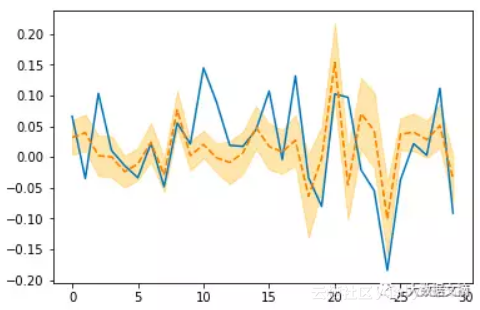30天的Pyro神经网络预测

for name in pyro.get_param_store().get_all_param_names():

print name, pyro.param(name).data.numpy()

import tensorflow as tf

sess = tf.Session()

with sess.as_default():

tf.global_variables_initializer().run()

dense_weights, out_weights = None, None

with sess.as_default():
for layer in model.layers:
if len(layer.weights) > 0:

weights = layer.get_weights()
if 'dense' in layer.name:

dense_weights = layer.weights.eval()
if 'out' in layer.name:

out_weights = layer.weights.eval()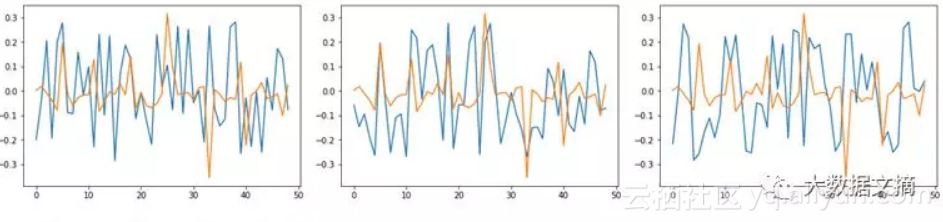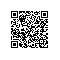使用钉钉扫一扫加入圈子
+ 订阅